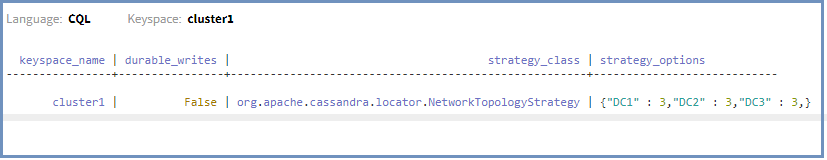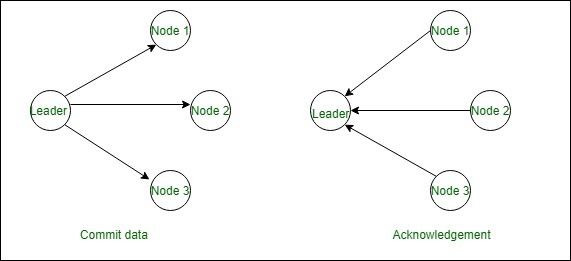Related Articles
Quorum Consistency in Cassandra
• Last Updated : 04 Feb, 2020

In this article, we will discuss how quorum consistency is helpful in Cassandra and how we can calculate it and also discuss how quorum consistency works.

What is Quorum Consistency?
Quorum consistency is consistency in Cassandra for high mechanism and to ensure that how many nodes will respond when we will define the read and write consistency in Cassandra. In Quorum consistency a majority of (n/2 +1) nodes of the replicas must respond.

In Quorum, we check the majority of replicas (which simply means that the number of replication factor). for example, if we have a replication factor of 3 in 2 data centers then how many their replicas will be there. so, there will be 6 and the majority is 4. (total_sum_replicas/2 + 1).

Generally, we use local quorum in most cases in which we need 2 nodes from the LOCAL DC to succeed when if there are three replicas each in 3 data centers.

Now, here we are just going to define the CQL query for the same. let’s have a look.

```CREATE KEYSPACE cluster1
with replication =  {'class' : 'NetworkTopologyStrategy',
'DC1': 3, 'DC2': 3, 'DC3': 3}
AND DURABLE_WRITES = false; ```

To verify the results used the following CQL query given below.

```SELECT *
FROM system_schema.keyspaces; ```

Output:How QUORUM is calculated ?
This is how we calculates quorum that simply means how many nodes will acknowledge.

`Quorum = (sum_of_replication_factors / 2) + 1 `

Quorum is equal to sum of replication factors division by 2 and added 1. It’s because to make it whole number.

Quorum consistency:
The sum of all the replication_factor settings for each data center is the sum_of_replication_factors.

```Total_sum_of_replication_factor = DC1_RF + DC2_RF + DC3_RF+ ... +DC_RF
```

How Quorum consistency works ?
If there are three nodes which is in majority and they have to respond in Quorum consistency then in below given diagram acknowledgement is showing that there are three nodes responding and at the time writing data then committed nodes showing that we committed our data into three nodes.In the above diagram if we are inserting data into table and RF =3 that means data will replicates on three nodes which will be available and this is shows in committed diagram. In case of acknowledgement diagram if we are reading data and there are total 5 nodes and apply the quorum consistency then (5/2 +1) nodes must responds. so, it is clear in diagram how three nodes are available and acknowledge for the same.

Attention reader! Don’t stop learning now. Get hold of all the important CS Theory concepts for SDE interviews with the CS Theory Course at a student-friendly price and become industry ready.

My Personal Notes arrow_drop_up
Recommended Articles
Page :